### The Brachistochrone Expanded and A Few Qeustions

This installment of “It’s Obvious. Not!” looks at:

Book: “Classical Dynamics of Particles and Systems”

Edition: third

Authors: Jerry B. Marion and Stephen T. Thornton

Publisher: Harcourt Brace Jovanovich

Pages: 176-178 (Chapter 5, Example 5.2)

Reading physics books, it often occurs to me that the authors must be aware of some patterns or 'rules of thumb' that the reader may not be privy to. Today's post expands a very truncated example from Marion and Thornton and hopefully clarifies it. This post also poses several questions in search of those patterns and rules mentioned above.

After explaining the calculus of variations and the importance of Euler's equation
Marion and Thornton follow up with a concrete example: the brachistochrone. The problem of the brachistochrone is to determine the path for a particle to move from point A to B under the influence of a constant force, (gravity for example), in the least amount of time. The 'least amount of time' phrase should key us that this is a good candidate for using Euler's Equation. In short order, the book determines that the path from point A to B can be described by the following integral equation: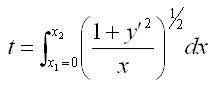the functional from this integral that should be used in Euler's equation is: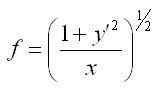as a reminder, Euler's equation is:The partial derivative of f with respect to y is 0. That means that the partial derivative of f with respect to y prime has to be constant. The authors decide to define that constant as one over the square root of two times the arbitrary quantity a.

By carrying out the partial differentiation from Euler's equation we arrive at, (using the chain rule of differentiation):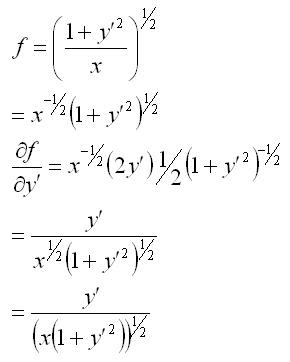The authors then decide to square the result to arrive at:This decision brings up the first question. The authors may have made this decision because they knew ahead of time that the final result for the path is a cycloid and the form of the partial differentiation shown above will lead them to that result. However, they offer no rationale as to their decision process.

Question 1: Is there a pattern that readers of the book should recognize that would lead them to square the result of the differentiation?

Next, the book solves the above equation for y, using the infamous expression 'This may be put in the form' and then stating the solution. Here are the stepsThose steps get us most of the way to the book's result. But, the last step is the tricky one and leads to the second question. The authors multiply the inside of the radical by one.Question Number 2: Is there something about the first result for y prime that should queue the reader to multiply the inside of the radical by x over x? Is it as simple as being familiar with integration tables and realizing that the multiplication will put the integrand in a familiar form?

From here, the book solves the integral by using a change of variable: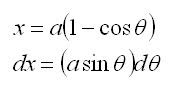This change of variable brings up the third question:

Question Number 3: Why did the authors chose this change of variables? It makes the solution come out as a very clean set of formulas parameterized on theta. Was there a pattern to the integral that experience reveals? Otherwise, the integral could have been worked out using integral tables and the answer would have been a rather messy expression involving an arcsin and more radicals.

Although the book performs the integral in one line, (they simply write down the answer), it's rather detailed. I'll work through the steps here.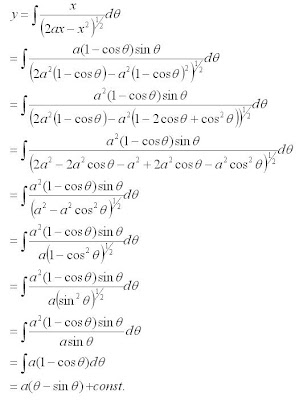These steps lead to one final question. If you followed the steps above, you may have come to the conclusion that:because these two terms cancel each other out in the initial quotient.

Question Number 4: Should the equality in the above expression have been obvious? Is there a simple way to look at this, (perhaps involving a trig identity), and immediately cancel the two terms in the quotient above?

I hope I've helped clear up how the book's solution leads from step to step. I believe the key to a solid understanding of physics lies partially in the ability to recognize what direction to move the solution in, in other words, in the thought processes that aren't revealed by the book.

Do you have any thoughts on how these steps could have been simplified? Do you know why the authors made the decisions they did? Do you know an even better way to solve this? Do you other questions about the solution? If so, please comment below!Anonymous said…
Thank you so much! Sorry this comment is coming 6 years after you wrote this, but I am so grateful for this post! M&T leaves so much for the reader, and it really does feel like they're privy to a lot of knowledge about the direction to take each derivation. Do you think this book an example of bad textbook writing?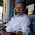Hamilton Carter said…
I'm glad the post was helpful! I think M&T just use a style that doesn't work for me. For others, like the professor that suggested it to me, their style probably work great. I've had far more luck with Landau and Lifshitz personally, but that's just because I enjoy their conciseness.

### Cool Math Tricks: Deriving the Divergence, (Del or Nabla) into New (Cylindrical) Coordinate Systems

The following is a pretty lengthy procedure, but converting the divergence, (nabla, del) operator between coordinate systems comes up pretty often. While there are tables for converting between common coordinate systems, there seem to be fewer explanations of the procedure for deriving the conversion, so here goes!

What do we actually want?

To convert the Cartesian nabla

to the nabla for another coordinate system, say… cylindrical coordinates.

What we’ll need:

1. The Cartesian Nabla:

2. A set of equations relating the Cartesian coordinates to cylindrical coordinates:

3. A set of equations relating the Cartesian basis vectors to the basis vectors of the new coordinate system:

How to do it:

Use the chain rule for differentiation to convert the derivatives with respect to the Cartesian variables to derivatives with respect to the cylindrical variables.

The chain rule can be used to convert a differential operator in terms of one variable into a series of differential operators in terms of othe…

### The Valentine's Day Magnetic Monopole

There's an assymetry to the form of the two Maxwell's equations shown in picture 1.  While the divergence of the electric field is proportional to the electric charge density at a given point, the divergence of the magnetic field is equal to zero.  This is typically explained in the following way.  While we know that electrons, the fundamental electric charge carriers exist, evidence seems to indicate that magnetic monopoles, the particles that would carry magnetic 'charge', either don't exist, or, the energies required to create them are so high that they are exceedingly rare.  That doesn't stop us from looking for them though!

Keeping with the theme of Fairbank and his academic progeny over the semester break, today's post is about the discovery of a magnetic monopole candidate event by one of the Fairbank's graduate students, Blas Cabrera.  Cabrera was utilizing a loop type of magnetic monopole detector.  Its operation is in concept very simpl…